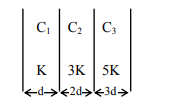# In the reported figure, a capacitor is formed by`
Question:

In the reported figure, a capacitor is formed by placing a compound dielectric between the plates of parallel plate capacitor. The expression for the capacity of the said capacitor will be :

(Given area of plate = A)1. $\frac{15}{34} \frac{\mathrm{K} \varepsilon_{0} \mathrm{~A}}{\mathrm{~d}}$

2. $\frac{15}{6} \frac{\mathrm{K}_{0} \mathrm{~A}}{\mathrm{~d}}$

3. $\frac{25}{6} \frac{\mathrm{K} \varepsilon_{0} \mathrm{~A}}{\mathrm{~d}}$

4. $\frac{9}{6} \frac{K \varepsilon_{0} A}{d}$

Correct Option: 1

Solution:

$\frac{1}{C_{\text {eff }}}=\frac{\mathrm{d}}{\mathrm{K} \in_{0} \mathrm{~A}}+\frac{2 \mathrm{~d}}{3 \mathrm{~K} \in_{0} \mathrm{~A}}+\frac{3 \mathrm{~d}}{5 \mathrm{~K} \in_{0} \mathrm{~A}}$

$\mathrm{C}_{\mathrm{eff}}=\frac{15 \mathrm{~K} \in_{0} \mathrm{~A}}{34 \mathrm{~d}}$